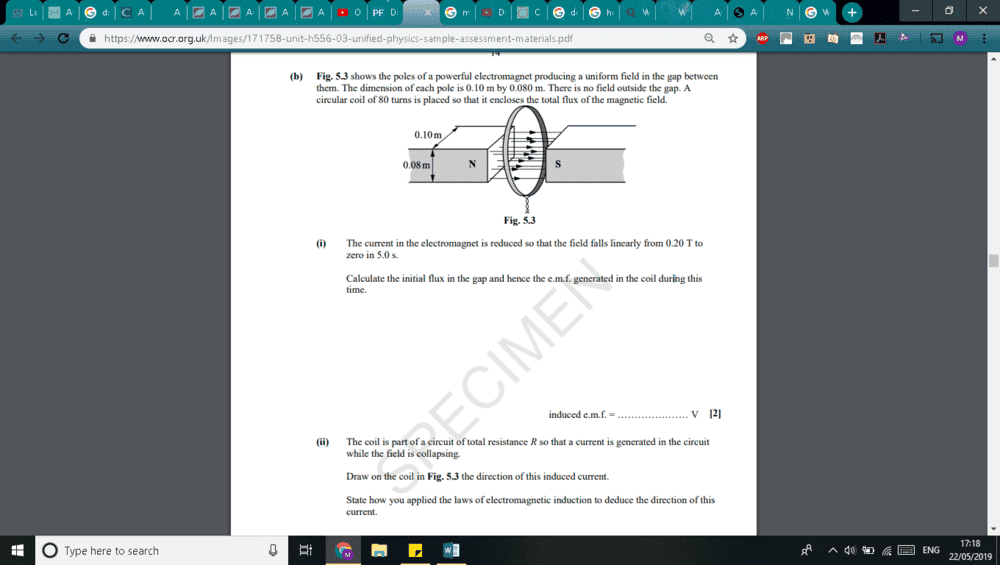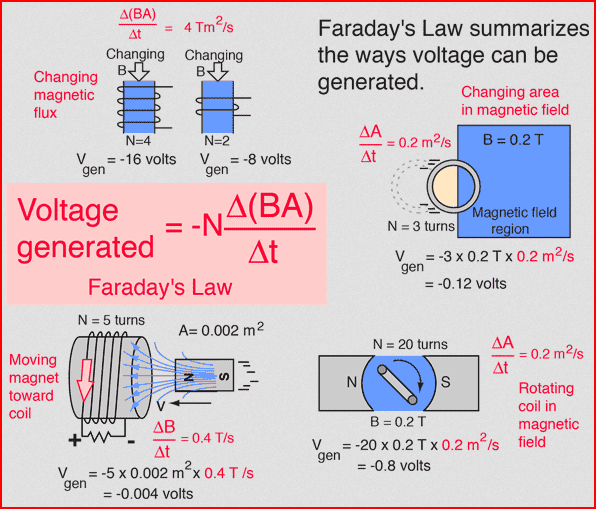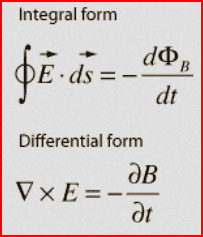# Direction of the induced current from an OCR paper -- concept question

• bonbon22

#### bonbon22

I am not sure in which direction this induced current would be I am assuming you have to use the dynmo rule or the right hand rule as this is the induced current, and the soft iorn core will cause a force to be produced in the coil which causes the current. If so in which direction would the force even be in? What does it mean when the magnetic field is collapsing , does this just tell me the object is leaving the area in which the magnetic field is acting in therefore the B value changes from X to zero, would that not induce a current if so because of faradays law ? Googling it tells me that the current would stop if magentic field collapses??
the answer is the south pole.Last edited:

It looks like you linked to a large PDF with lots of exam questions. Can you just post a screenshot of the single question you are asking about? Thanks (use the Windows Snipping Tool or use Alt-PrintScreen to capture the image, and paste it into a reply here)

Also, there are indeed Relevant Equations to use. Can you take some guesses at which equations apply?

It looks like you linked to a large PDF with lots of exam questions. Can you just post a screenshot of the single question you are asking about? Thanks (use the Windows Snipping Tool or use Alt-PrintScreen to capture the image, and paste it into a reply here)

Also, there are indeed Relevant Equations to use. Can you take some guesses at which equations apply?
done

•berkeman
Thanks!
Also, there are indeed Relevant Equations to use. Can you take some guesses at which equations apply?
And what is the equation that relates the change in flux through a loop to the voltage induced in the loop by that change?

•bonbon22
Thanks!

And what is the equation that relates the change in flux through a loop to the voltage induced in the loop by that change?

Answer which question? You should use that equation to calculate the EMF and then the current for the 2nd part of the question. They have drawn the direction of the flux, but say that it is decreasing, so which way does the derivative of the vector flux point?

http://hyperphysics.phy-astr.gsu.edu/hbase/electric/imgele/farlaw.pngAnswer which question? You should use that equation to calculate the EMF and then the current for the 2nd part of the question. They have drawn the direction of the flux, but say that it is decreasing, so which way does the derivative of the vector flux point?

http://hyperphysics.phy-astr.gsu.edu/hbase/electric/imgele/farlaw.png
View attachment 244005
Interesting , so if flux is decreasing its pointing in the opposite direction? So south to north? So need to use right hand rule here?

also in that image does emf induced ever become negative in a rotating coil as Area is not a vector ?

Interesting , so if flux is decreasing its pointing in the opposite direction? So south to north? So need to use right hand rule here?
When the flux is pointing N-S and is decreasing, then the change in flux is pointing S-N. It's best to think of the flux as a function of time as a vector field. Also be careful about the negative sign in Faraday's Law...
also in that image does emf induced ever become negative in a rotating coil as Area is not a vector ?
Area is not a vector, but the Normal to a surface is a vector...

Have you learned the calculus version of Faraday's law yet? E and B are vectors, and the B is calulated by integrating the flux vector over the surface area that the coil surrounds...

http://hyperphysics.phy-astr.gsu.edu/hbase/electric/imgel2/maxw13.gifWhen the flux is pointing N-S and is decreasing, then the change in flux is pointing S-N. It's best to think of the flux as a function of time as a vector field. Also be careful about the negative sign in Faraday's Law...

Area is not a vector, but the Normal to a surface is a vector...

Have you learned the calculus version of Faraday's law yet? E and B are vectors, and the B is calulated by integrating the flux vector over the surface area that the coil surrounds...

http://hyperphysics.phy-astr.gsu.edu/hbase/electric/imgel2/maxw13.gif
View attachment 244007
i have not, I am doing a level physics , which does not have any integration differentiation ..

Okay so now i know that the flux is decreasing so the change in Flux is moving in the south to north direction, i understand that now, and the rate of change of flux is the emf so I am guessing that the emf acts in the same direction as the current?

i have not, I am doing a level physics , which does not have any integration differentiation ..

Okay so now i know that the flux is decreasing so the change in Flux is moving in the south to north direction, i understand that now, and the rate of change of flux is the emf so I am guessing that the emf acts in the same direction as the current?
The EMF generates current in the same direction. But again, be careful of the minus sign in Faraday's Law...